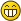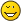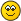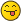Describing the beta method using fractional linear transformationsJmsNxnUltimate FellowPosts: 1,214 Threads: 126 Joined: Dec 2010 08/05/2022, 03:35 AM (This post was last modified: 08/05/2022, 04:04 AM by JmsNxn.) As Bo has started to talk a lot about linear fractional iterations, I thought I'd throw in my hat on some results I know about Linear fractional relations, and how they compare to what Bo is talking about. I'd like to put a little disclaimer though, that these iterations are wildly different beasts than Tetration--and wildly different than Bo's regular iterations. But with that out of the way, we can get started. ------------------------ INFINITE COMPOSITIONS Infinite compositions behave a lot differently for automorphisms of $\widehat{\mathbb{C}}$. We can remember that an automorphism of $\widehat{\mathbb{C}}$ looks like: $\mu(z) = \frac{az + b}{cz + d}\\$ Which, as Bo has pointed out, looks like a matrix applied to the $(x,y)$ coordinate--$z=x+iy$: $\begin{pmatrix} a & c\\ b & d \end{pmatrix}$ So long as the determinant $\det(\mu) \neq 0$--i.e $ad - bc \neq 0$--this is an automorphism of $\mathbb{R}^2$. Now, this tends to be diagonalizable/ then iterated through its eigenvalues from the diagonalization; I'm not going to do that. That's a horse that's been beaten one too many times. Let's instead, write: $\mu_n(z) = \frac{a_nz + b_n}{c_nz + d_n}\\$ Such that each matrix satisfies $\det(\mu_n) \neq 0$. We are going to look at the function: $U_N(z) = \Omega_{n=1}^N \mu_n(z)\bullet z = \mu_1(\mu_2(...\mu_N(z)))\\$ And ask where it converges as $N\to\infty$. By which, if I proclaim that: $\sum_{n=1}^\infty \det(\mu_n - I) < \infty\\$ For the identity matrix $I$. Then the above function converges compactly on $\widehat{\mathbb{C}}$. This follows from a proof that's 4 years gone by now on how infinite compositions converge. I had originally proved this for entire functions; and it becomes much more placated for linear fractional transformations. The more natural way I would write this, is if for $K \subset \widehat{\mathbb{C}}$ is compact: $\sum_{n=1}^\infty \sup_{z \in K}|\mu_n(z) - z| < \infty\\$ Then $\lim_{N\to\infty} U_N(z) = U(z)$ everywhere on $\widehat{\mathbb{C}}$. When we talk about the Beta method, we are not talking about something that looks like this. We are talking about a very different beast. We are asking instead, that $det(\mu_n) \to 0$ as $n \to \infty$ This introduces the formula: $\sum_{n=1}^\infty \sup_{z\in K} |\mu_n(z)| < \infty$ The function $U$ in this scenario always converges to a CONSTANT in z. So it is holomorphic in $z$, but it's constant. The beta method then asks, that instead we write: $\mu_n(z) = \mu(-n,z)\\$ Where $\mu(s,z)$ is holomorphic in $s$ but maps $\widehat{\mathbb{C}} \to \widehat{\mathbb{C}}$. Then, if, on compact sets in $s$, along with compact sets in $z$ we have: $\sum_{n=1}^\infty \sup_{s\in S,z\in K} |\mu(s-n,z)| < \infty$ Then we've created a beta function which looks like: $U(s) = \Omega_{n=1}^\infty \mu(s-n,z)\bullet z\\$ Which satisfies: $U(s+1) = \mu(s,U(s))\\$ Since we've allowed for poles, and poles are natural, this function $U(s)$ maps $\widehat{\mathbb{C}} \to \widehat{\mathbb{C}}$, so long as the summation in $s$ converges for the extended plane. Which isn't as scary as it seems. ---------------------------- Reinventing the beta function Let's take Bo's iteration of: $\mu(z) = \frac{1}{1+z}\\$ Which he described using Fibonacci's sequence. Let's play a bit looser though. Let's write, for $\Re(\lambda) > 0$: $\mu(s,z) = \frac{1}{(1+e^{-\lambda s})(1+z)}\\$ Then this function satisfies: $\sum_{n=1}^\infty \sup_{s\in S,z\in K} \left|\frac{1}{(1+e^{\lambda(j- s)})(1+z)}\right| < \infty\\$ This is obvious away from $z \approx -1$, we have to do a change of variables near $-1$. So, additionally, where there are poles, we can change a different coordinate space and the sum converges. The benefit of working with a perfect Riemann surface $\widehat{\mathbb{C}}$. But either way, this is no different than: $\sum_{n=1}^\infty |\det(\mu(s-n))| < \infty\\$ So when we take the infinite composition we get: $U(s) = \Omega_{n=1}^\infty \mu(s-n,z)\,\bullet z = \mu(s-1,\mu(s-2,...\mu(s-n,...)))\\$ This function satisfies: $\frac{1}{1+U(s)} \cdot \frac{1}{1+e^{-\lambda s}} = U(s+1)\\$ And you wouldn't be that hard pressed to prove this is a meromorphic function in $\widehat{\mathbb{C}}$. ----------------------------------- So what does this have to do with bo? Since $\mu(+\infty,z) = \mu(z) = \frac{1}{1+z}$, then this is a bijection of $\widehat{\mathbb{C}}$. Then $\mu^{-1}(z) = h(z) = \frac{1-z}{z}$. So the beta method for all of bo's fancy iterations is precisely: $\lim_{n\to\infty} h^{\circ n}(U(s+n)) = F_\lambda(s)\\$ But in order for this to converge, we write: $F_\lambda(s) = U(s) + \tau(s)\\$ Where we're taking advantage of the asymptotic formula: $h(U(s+1)) = U(s) + O(e^{-\lambda s})\\$ That these objects are asymptotically equivalent (in a fairly strong manner) to the iterations you are writing. Note, that this iteration is periodic with period $2\pi i/\lambda$. It will by its nature have a measure zero "no-go" zone, but it will definitely converge everywhere else. I am mostly just making this post to describe the $\beta$ method, but using linear fractional transformations. I'm doing this, I hope for everyone's benefit. And especially, because for linear fractional transformations, the beta method is much more straight forward. So, as Bo is drawing a parallel using LFTs, I am drawing a parallel using LFTs. And I am doing so specific to what makes the beta method interesting. Additionally, I'm hoping to explain this construction more simply to Bo, who I don't think has grasped the beta method and how it works. How am I doing, boEDIT! Also, much of my work on stuff like this is not published in a peer reviewed manner. But I'm fairly well known at U of T for a lot of good work, and much of my work has been peer reviewed by professors at U of T. So it's not all coming out of my ass like your regular poster. A bit is coming outta my ass; but I've been "peer reviewed" on a lot of my work at u of t.bo198214Administrator Posts: 1,624 Threads: 103 Joined: Aug 2007 08/05/2022, 04:23 PM (08/05/2022, 03:35 AM)JmsNxn Wrote: So what does this have to do with bo?I feel a bit uncomfortable if you do this personally for meQuote:Additionally, I'm hoping to explain this construction more simply to Bo, who I don't think has grasped the beta method and how it works. How am I doing, boEDIT! Also, much of my work on stuff like this is not published in a peer reviewed manner. But I'm fairly well known at U of T for a lot of good work, and much of my work has been peer reviewed by professors at U of T. So it's not all coming out of my ass like your regular poster. A bit is coming outta my ass; but I've been "peer reviewed" on a lot of my work at u of t. Sounds all pretty solid to me. Why don't you going to publish? Proven convergence of methods to calculate crescent iteration is a rare thing. Though I could imagine your proofs could become a bit wobbly if it is about to sending the period to infinity. I just remember Kouznetsov's solution is asymptotically periodic, did you consider this already? Yeah this brings the beta method much closer to me. Though it is also a psychological thing: If someone says: read this article and tell me, its by no way as inviting than developing it in a dialogue. That's why I always would people encourage to post and explain things (so to say interactively) in the forum than just referencing something that they wrote somewhere. On the other hand you are really lucky that I am pressing more time from myself to spend on the forum than is good for me!DanielLong Time FellowPosts: 262 Threads: 88 Joined: Aug 2007 08/05/2022, 10:48 PM (08/05/2022, 04:23 PM)bo198214 Wrote: ... On the other hand you are really lucky that I am pressing more time from myself to spend on the forum than is good for me! Yes, I noticed you are posting regularly. Please take care of yourself, but the postings are awesome and invaluable. Mathematicians who share how they think is rare but a blessing. DanielJmsNxnUltimate FellowPosts: 1,214 Threads: 126 Joined: Dec 2010 08/07/2022, 08:39 AM (08/05/2022, 04:23 PM)bo198214 Wrote: (08/05/2022, 03:35 AM)JmsNxn Wrote: So what does this have to do with bo?I feel a bit uncomfortable if you do this personally for meBo, you are a legend. I just want you to understand where I'm coming from in my descriptions. If you call me out I take it as a badge of honourGottfriedUltimate FellowPosts: 900 Threads: 130 Joined: Aug 2007 08/07/2022, 09:39 AM (08/07/2022, 08:39 AM)JmsNxn Wrote: Bo, you are a legend. I just want you to understand where I'm coming from in my descriptions. If you call me out I take it as a badge of honourCompliments, finetuned like this, are always a nice ingredient of forums like this. Never forget!Gottfried Helms, KasselJmsNxnUltimate FellowPosts: 1,214 Threads: 126 Joined: Dec 2010 08/07/2022, 12:15 PM (This post was last modified: 08/07/2022, 02:46 PM by JmsNxn.) (08/07/2022, 09:39 AM)Gottfried Wrote: (08/07/2022, 08:39 AM)JmsNxn Wrote: Bo, you are a legend. I just want you to understand where I'm coming from in my descriptions. If you call me out I take it as a badge of honourCompliments, finetuned like this, are always a nice ingredient of forums like this. Never forget!Gottfried, I imagine you could describe the lackluster Matrix representation I made in this post. I just know that the identification is the same--I think much more classical analyst though (everything is a taylor series; euler manipulation; mixed with hard german "normal summation"). I suck at matrices, so I'm sure I misexplained something to do with the 2x2 matrix interpretation, lol. (08/05/2022, 04:23 PM)bo198214 Wrote: Sounds all pretty solid to me. Why don't you going to publish? Proven convergence of methods to calculate crescent iteration is a rare thing. Though I could imagine your proofs could become a bit wobbly if it is about to sending the period to infinity. I just remember Kouznetsov's solution is asymptotically periodic, did you consider this already? Also, bo. Covid happened. I was offered the beginnings of a PHD program at U of T--and then bam! Covid. So I couldn't go downtown toronto, I couldn't really see the university. On top of me living with my elderly parents. So I kind of got shoehorned out of taking the scholarship they offered me. I will probably go back into it, but it's up in the air since covid. Like, they offered me a good ride PHD 2 years ago, does that still carry over? So I'm kind of in academic limbo right now, lol. But I was offered a PHD program at U of T, I just fumbled a bit, and then Covid happened and there was no way with all that chaos. But I do plan to try to work something out again, lol. But a bunch of mathematicians and physicists know me at U of T for a bunch of dumb shit I've done. So I have a fairly good reputation lolFor example, I was an accepted undergraduate in a graduate level class on analytic number theory, and I got the highest mark in the class out of even the graduate level students. Things like that.... So I'm not all full of shit, boIt's also important to note, if we're being honest. I do not have my undergraduate. And I was offered a 3 year plan to get my phd without an undergraduate degree. I mostly don't have my undergraduate because I've had a lot of personal problems in my life; but I've always found love in math. And U of T has been really good to me. And I got in good with professors, and I showed my true colours. And I kind of got special treatmentBut the only thing not giving me my undergraduate degree is the credit requirements--I have graduate level credits in math; but I don't have all the year 1 year 2 credits you need. (So for example I have a bunch of 4th year calculus credits, but I don't have the initial year 1 calculus credit so it fucks everything up with the degree they hand out, lmao) What ever, thought I'd be honest to you bo. I'm looking to go back, and hopefully I can try and get the same arrangement, 3 year PHD kind of thing. I'm only 30 years old, I figure I'm still young. I'm sure many people get their PhD at 34. My brother is nearly 40 still working on his phd thesis in korean history, lol. He's gotta write that shit in korean too! God I don't envy him, lol. But long story short, that's why I don't care about hard publishing my work. No one gives a shit til you have a PHD. I've been offered publishing in low level journals but I always turn it down. Go big or go home. I primarily publish my work on arxiv, and that has served me well. If or when I get my PHD then I'll think about proper journals--like your work. Or any strong work. I'm mostly publishing notices and descriptions of what's going on. ALSO You said Kouznetsov asymptotically periodic. There is a proof somewhere that $b = \eta$ has not quite an almost periodic, so it's not almost periodic, but you can do something to put it in the almost periodic space. Is this what you're talking about Kouznetsozv asymptotically periodic? Sorry when I hear that I go straight to Hilbert spaces--that was also much more what I was known for at U of T. Hilbert spaces and analytic number theory stuff. lol. « Next Oldest | Next Newest »

 Possibly Related Threads… Thread Author Replies Views Last Post ChatGPT checks in on fractional iteration. Daniel 0 54 05/17/2023, 01:48 PM Last Post: Daniel The ultimate beta method JmsNxn 8 442 04/15/2023, 02:36 AM Last Post: JmsNxn Bridging fractional iteration and fractional calculus Daniel 8 377 04/02/2023, 02:16 AM Last Post: JmsNxn greedy method for tetration ? tommy1729 0 164 02/11/2023, 12:13 AM Last Post: tommy1729 tommy's "linear" summability method tommy1729 15 912 02/10/2023, 03:55 AM Last Post: JmsNxn Fractional Integration Caleb 11 736 02/10/2023, 03:49 AM Last Post: JmsNxn another infinite composition gaussian method clone tommy1729 2 279 01/24/2023, 12:53 AM Last Post: tommy1729 Semi-group iso , tommy's limit fix method and alternative limit for 2sinh method tommy1729 1 340 12/30/2022, 11:27 PM Last Post: tommy1729 Discussing fractional iterates of $f(z) = e^z-1$ JmsNxn 2 480 11/22/2022, 03:52 AM Last Post: JmsNxn [MSE] short review/implem. of Andy's method and a next step Gottfried 4 748 11/03/2022, 11:51 AM Last Post: Gottfried

Users browsing this thread: 1 Guest(s)